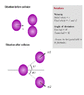# Find the coefficient of restitution

Gold Member

## Homework Statement

Two equal balls are in contact on a table. A third equal ball strikes them simultaneously and remains at rest after the impact. Show that the coefficient of restitution is 2/3.
I have attached an image for clarity of problem. Please open it.

## Homework Equations

Conservation of Momentum

## The Attempt at a Solution

Let the mass of balls be m and coefficient of restitution be e.

$\frac{v_{2}}{vcosθ_{2}} = e$

Also,
$\frac{v_{1}}{vcosθ_{1}} = e$

Applying Conservation of Momentum along X-axis

$v=v_{1}cosθ_{1}+v_{2}cosθ_{2}$
Along Y- axis
$v_{1}sinθ_{1}=v_{2}sinθ_{2}$

Substituting the value of v1 and v2 in the above equation
$evcosθ_{1}sinθ_{1}=evcosθ_{2}sinθ_{2}$
$cosθ_{1}sinθ_{1}=cosθ_{2}sinθ_{2}$

Multiplying 2 on both sides
$sin2θ_{1}=sin2θ_{2}$
Rearranging and simplifying
$2cos(θ_{1}+θ_{2})sin(θ_{1}-θ_{2})=0$
$θ_{1}+θ_{2}=\frac{∏}{2}$
$θ_{1}-θ_{2}=0$

$θ_{1}=θ_{2} and θ_{1} = \frac{∏}{4}$

Now substituting the value of θ1 in equation of momentum along X-axis

$1=e(cos^{2}θ_{1}+cos^{2}θ_{2})$
$e=\frac{1}{cos^{2}θ_{1}+cos^{2}θ_{2}}$
$e=\frac{1}{2cos^{2}θ_{1}}$
$e=1$

What's wrong here?#### Attachments

•PF1.gif
19.4 KB · Views: 531

klawlor419
I will say first to reconsider your expressions for the coefficients of restitution. Also check the momentum conservation. Furthermore it may help to consider energy conservation as well. As this gives a further restraint on the values in the problem.

Gold Member
I will say first to reconsider your expressions for the coefficients of restitution. Also check the momentum conservation. Furthermore it may help to consider energy conservation as well. As this gives a further restraint on the values in the problem.
energy conservation principle can't be applied here because the collision is not elastic. I also suspect something wrong in my expressions for coefficient of restitution. But I can't figure out what is it. Also I don't think that momentum conservation equations are wrong.

klawlor419
Well if it is indeed an inelastic collision than you are correct. It was not specified in your problem statement. You're off by a minus sign in one of your momentum conservation equations. The definition of the coefficient of restitution is fractional value of the ratio of speeds before and after a collision. You should be able to find the error by just considering this definition and looking at your expressions for, e.

shutdo
If the 3 balls are identical and the collisions occur simultaneously. You can immediately deduce that.
The lines of impacts of both collisions pass through the centres and therefore,
$$\theta_1=\theta_2=\frac{\pi}{6}\\ and\\ v_1=v_2$$
Then your answer is therefore $e=\frac{2}{3}$

#### Attachments

Last edited:
Gold Member
If the 3 balls are identical and the collisions occur simultaneously. You can immediately deduce that.
The lines of impacts of both collisions pass through the centres and therefore,
$$\theta_1=\theta_2=\frac{\pi}{6}\\ and\\ v_1=v_2$$
Then your answer is therefore $e=\frac{2}{3}$

Hey, why didn't I think it earlier! Thank You.